Notebook

# Quantpedia Series: Reversal in the PEAD¶

By Matt Lee

This research is published in partnership with Quantpedia, an online resource for discovering new trading ideas.

You can view the full Quantpedia series in the library along with other research and strategies.

Whitepaper author: Jonathan A. Milan

Whitepaper source: https://www.aeaweb.org/conference/2014/retrieve.php?pdfid=731

## Abstract¶

From Quantpedia:

>The post-earnings announcement drift (the tendency of stocks to drift in a direction of earnings announcement surprise during next quarter) is a well known effect many times analyzed in an academic literature. However recent research speculates that maybe it is known too much. Arbitrageours started to expoit this anomaly and it seems that effect reversed in the most liquid stocks. Research paper by Milian shows that stocks which had the worst return during past earning announcement deliver substantially better return during the days around next earnings announcement. Classical PEAD (post-earnings announcement drift) literature examines mainly quarterly returns therefore it is probable that PEAD still holds. However Milian's work shows a way how to profit from traders over-reaction to a classical anomaly.

## Introduction¶

Recent research speculates that the PEAD has been reversed in recent years, due to the overcrowding of arbitrageours invested in PEAD strategies. In other words, firms that provided the biggest positive earnings announcement surprise have significant negative returns shortly after their subsequent earnings announcement.

This can be attributed to the idea that investors know they typically underreact to earnings announcement news (the underlying explanation for the PEAD). Thus, by compensating for their underreaction, they overreact to substantial surprises in earnings announcement news, positioning themselves in alignment with the expectation of the PEAD effect. When the next learnings announcement comes, the overcrowding of investors pushes the market beyond efficient, resulting in the correction of investor sentiment and a negative correlation for firm's earnings news in the following days.

Milian's paper found evidence of the PEAD reversal over a sample period from 2003 - 2010.

In this notebook, I continue Milian's study over a sample period from 2011 - 2016. I find similiar results to the paper, with a couple adjustments, confirming that the PEAD reversal effect has continued over recent years.

You can navigate the rest of this notebook as follows:

</a>

# Methodology and Sample¶

>I've written a separate article which goes in depth about how I generated the data used in this study. Check it out here (or read through the code on the bottom of the page).

The data in our sample consists of stocks in the Q500US over the period of 2011- 2016

In :
start = pd.Timestamp("1-05-2011")
end = pd.Timestamp("09-25-2016")
stock_data = create_data(Q500US(), start, end, 12)
price_data = get_pricing(stock_data['sid'].unique(), start - pd.Timedelta('120d'), end + pd.Timedelta('10d'), fields='open_price')

In :
stock_data = compute_returns(stock_data, price_data)


Here's a peek of the data that I've generated. All stocks in this dataset are from the Q500US - since Quantopian currently doesn't support options data, we use the Q500US as a proxy for stocks with active options trading (as the paper suggests)

In :
stock_data.head()

Out:
Current Day sid ADV Percentile Current Price Earnings Announcement LagEaSurp Previous Earnings Announcement Sector Quarter LagEaSurp Decile ... T 7 T 8 T 9 T 10 T 11 T 12 T 13 T 14 T 15 LagEaRet Decile
0 2011-01-05 00:00:00+00:00 Equity(22140 [MON]) 88 68.77 2011-01-06 -50.00 2010-10-06 101 Q12011 0.0 ... 0.081718 0.074197 0.075788 0.029216 0.017211 0.030662 0.027770 0.045415 0.068123 7
1 2011-01-07 00:00:00+00:00 Equity(2 [AA]) 90 16.36 2011-01-10 50.00 2010-10-07 101 Q12011 9.0 ... -0.005471 -0.032827 -0.023100 -0.035258 -0.007295 -0.006687 0.009726 0.005471 -0.016413 9
2 2011-01-07 00:00:00+00:00 Equity(24829 [APOL]) 43 39.45 2011-01-10 1.55 2010-10-13 205 Q12011 3.0 ... 0.089209 0.072530 0.093252 0.071519 0.056609 0.067475 0.065959 0.070003 0.036897 0
4 2011-01-13 00:00:00+00:00 Equity(5117 [MTB]) 27 87.39 2011-01-14 4.96 2010-10-20 103 Q12011 5.0 ... -0.011004 -0.001719 -0.013641 -0.004700 -0.008826 -0.006534 -0.001719 -0.009972 -0.005044 1
5 2011-01-13 00:00:00+00:00 Equity(25006 [JPM]) 98 44.72 2011-01-14 10.99 2010-10-13 103 Q12011 7.0 ... -0.002893 0.005785 0.000890 0.002448 -0.011794 0.005785 0.018914 0.012016 0.001335 3

5 rows × 28 columns

So - for each day we take measurements about the stocks which have an earnings announcement the next day.

The most notable variables we keep track of are:

1. LagEaSurp: A measurement of previous earnings announcement surprise by difference between analyst forecasts and actual values
2. LagEaRet: A measurement of the 2 day returns following the previous earnings announcement (another indicator of earnings surprise)
3. LagEaSurp Decile: The Decile which this stock's LagEaSurp falls in
4. LagEaRet Decile: The Decile which this stock's LagEaRet falls in

We also keep track of the forward returns data for each stock

1. T (day): The returns after a certain number of days

Some supplementary variables we track are:

1. ADV Percentile: Average Dollar Volume percentile
2. Sector: Market Sector

We will experiment with these supplementary variables later once we've done our inital checks. Certain sectors could be prone to this reversal, ADV, and we might want to experiment with the hold time

</a>

# Empirical Results¶

## Results Overview¶

### Milian (2003 - 2010)¶

Milian found compelling evidence of the PEAD reversal over a sample period of 2003 - 2010

Over 2003-2010 he found that:

• Stocks in Lowest decile of LagEaRet: 1.51% average 2 day return after the earnings announcement
• Stocks in Highest decile of LagEaRet: -.08% average 2 day return after the earnings announcement
• Stocks in Lowest decile of LagEaSurp: .68% average 2 day return after the earnings announcement
• Stocks in Highest decile of LagEaSurp: -1% average 2 day return after the earnings announcement

Spearman Correlation Coefficients:

• LagEaRet and 2 day returns after ea: -.04
• LagEaSurp and 2 day returns ea: -.03

In short, he found some correlations between both LagEaRet and LagEaSurp with 2 day returns after earnings announcements, although LagEaRet was the stronger indicator of the two.

### Quantopian OOS (2011-2016)¶

We found that a 9 Day and 5 day hold period for LagEaret picks and LagEaSurp picks yielded more returns that a 2 day hold period.

For our hold periods we found:

• Stocks in Lowest decile of LagEaRet: 1.47% average 9 day return after the earnings announcement
• Stocks in Highest decile of LagEaRet: - .3% average 9 day return after the earnings announcement
• Stocks in Lowest decile of LagEaSurp: .99% average 5 day return after the earnings announcement
• Stocks in Highest decile of LagEaSurp: -.12% average 5 day return after the earnings announcement

Spearman Correlation Coefficients:

• LagEaRet and 10 day returns: -.052
• LagEaSurp and 5 day returns: -.04

For a 2 day hold period, we found less compelling results, the lowest deciles of LagEaSurp and LagEaRet produced returns of .65 and lower spearman coefficients, suggesting that the model in the paper is deprecated.

So our OOS seems to show that this PEAD reversal is indeed still happening in recent years, albeit with a slightly adjusted strategy.

## Results Evaluation Process¶

I tested the PEAD reversal strategy outlined by the paper on the Q500 universe from 2011 to 2016. The Q500 was chosen since the paper recommended stocks with active-traded options, and the Q500 is a decent proxy for that.

From now on T refers to the day before the Earnings Announcement (the day which we will hypothetically purchase stocks)

Remember that LagEaSurp refers to the earnings surprise of the previous earnings announcement. LagEaRet is the 2 day returns after the previous earnings_announcement. Both are measures of earnings surprise.

We use these measures, and group them by decile to see whether the hold any predictive power over the returns following the upcoming announcement (which occurs at T + 1). Decile 0 corresponds to the lowest LagEaRet and LagEaSurp - the most negative previous earnings surprises, and Decile 9 corresponds to the most positive previous earnings surprises.

First, I take a look at the average returns by decile of both LagEaSurp and LagEaRet, from T to T + 3 (two days after the earnings announcement), to see whether Milian's PEAD reversal has continued over recent years.

In :
dec_plot(stock_data, "LagEaSurp Decile", "T 3")
dec_plot(stock_data, "LagEaRet Decile", "T 3")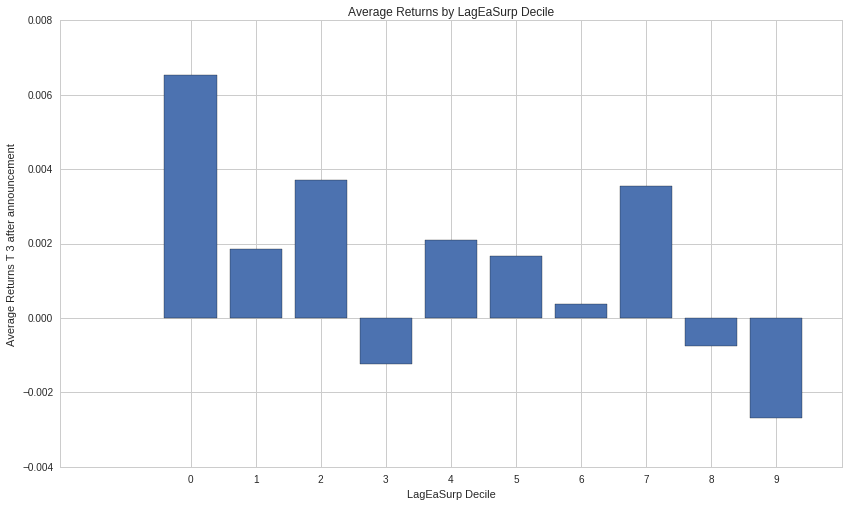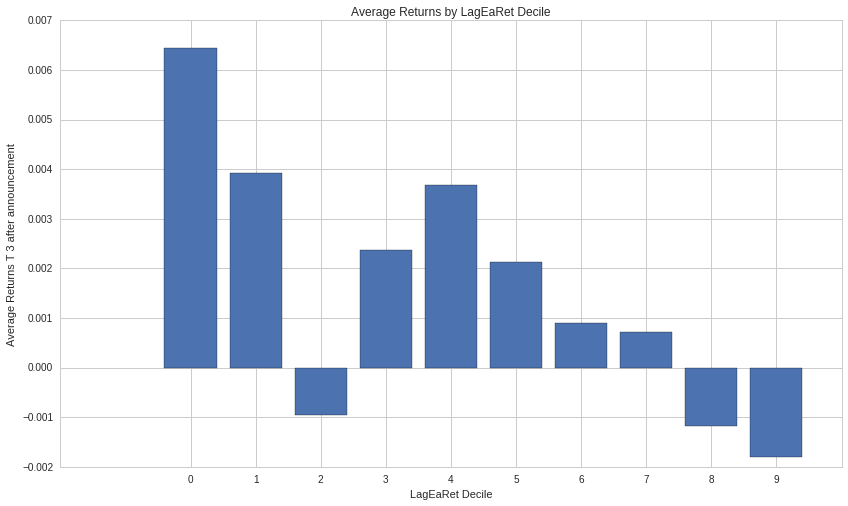In :
print ("Average Lowest LagEaRet Decile T + 3 Performance", stock_data[stock_data["LagEaRet Decile"] == 0]["T 3"].mean())
print ("Average Lowest LagEaSurp Decile T + 3 Performance", stock_data[stock_data["LagEaSurp Decile"] == 0]["T 3"].mean())
print ("Average Highest LagEaRet Decile T + 3 Performance", stock_data[stock_data["LagEaRet Decile"] == 9]["T 3"].mean())
print ("Average Highest LagEaSurp Decile T + 3 Performance", stock_data[stock_data["LagEaSurp Decile"] == 9]["T 3"].mean())

('Average Lowest LagEaRet Decile T + 3 Performance', 0.006444344470342162)
('Average Lowest LagEaSurp Decile T + 3 Performance', 0.006539099737748851)
('Average Highest LagEaRet Decile T + 3 Performance', -0.0017950411209190188)
('Average Highest LagEaSurp Decile T + 3 Performance', -0.0026976077702601763)


So far, the results look decent and relatively aligned with the paper. We see that the lowest decile average cumulative returns from T - 1 (day before earnings) to T + 2 are .65 % for LagEaSurp and .64% for LagEaRet, and the highest decile returns are -.26 % and -.17% in a two day window for LagEaSurp and LagEaRet respectively. These results are less than the original paper found, but are still a compelling result

It seems that LagEaRet and LagEaSurp give similiar returns predictions for the highest and lowest decile. Next, we can plot the spread over for both indicators over our sample period. The spread is the returns of the lowest decile - returns of the highest decile and represents our average 2 day returns over the period

In :
plot_spread_year(stock_data, "LagEaRet Decile", 'T 3')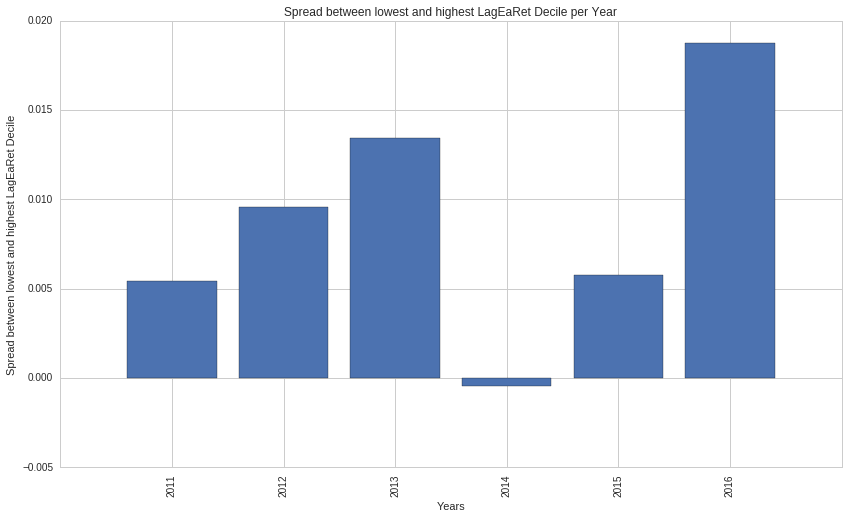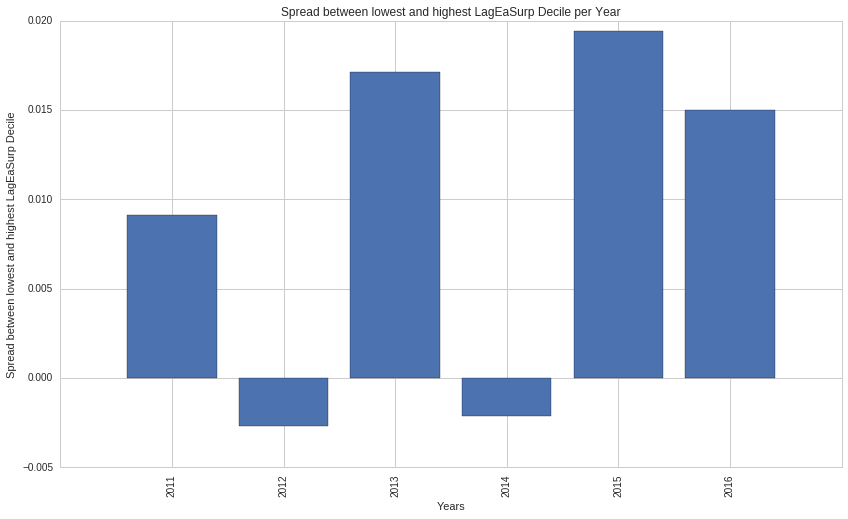Overall, the strategy using LagEaRet seems to be more consistent and profitable than the LagEaSurp.

Since it seems that the lowest decile is driving most of our returns, it would be interesting to see the quarterly performance of the lowest decile for LagEaRet and LagEaSurp

In :
low_dec_performance(stock_data, "LagEaRet Decile", 'T 3')
low_dec_performance(stock_data, "LagEaSurp Decile", 'T 3')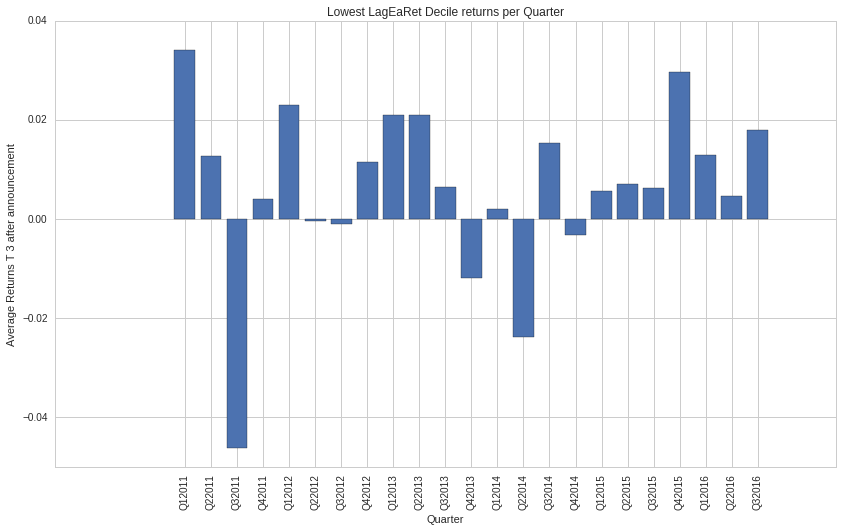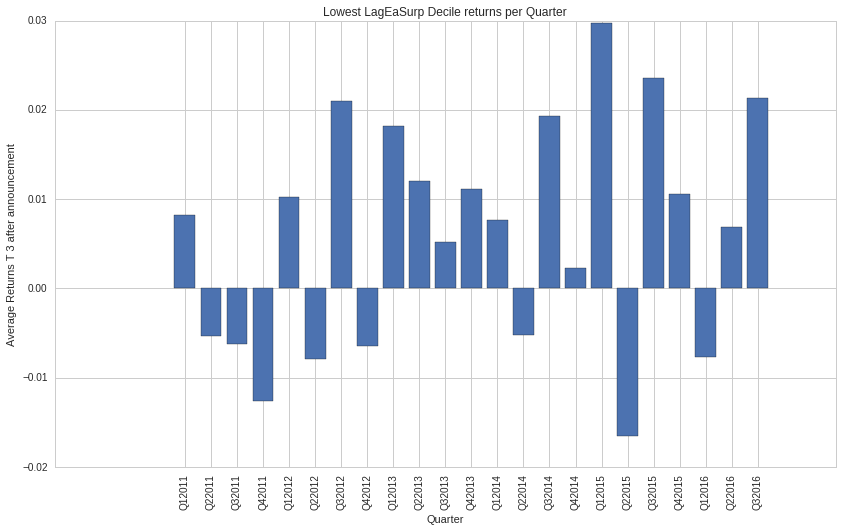Again, LagEaRet indicator is more consistent, with a net negative for 4 quarters in the sample versus the 8 quarters from LagEaSurp

Next, we can look at the cumulative returns by decile over the earnings announcement time period

In :
plot_cum_rets(stock_data, "LagEaRet Decile")
plot_cum_rets(stock_data, "LagEaSurp Decile")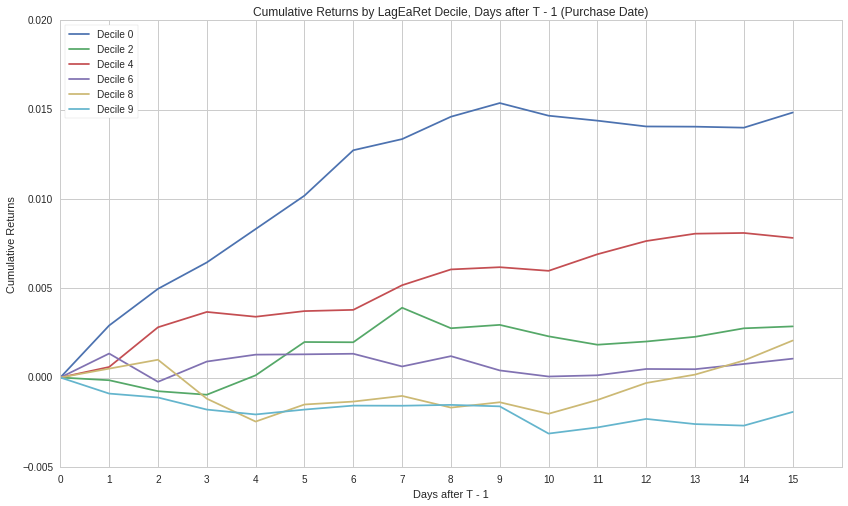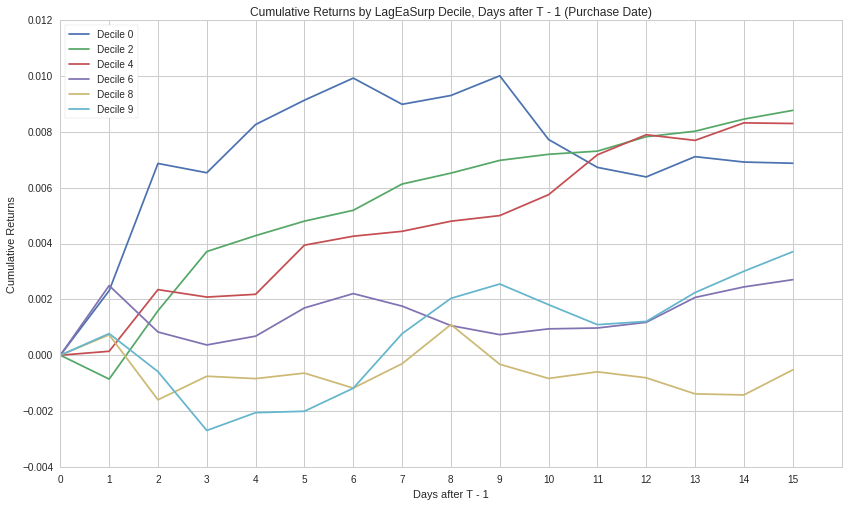Here, the x value of 1 represents the returns on the open of the earnings announcement. We can see the stock price is drastically adjusted by the day after the earnings announcement, and continues to move in the opposite expectation of the PEAD.

What's really interesting is the continuous spike of Decile 0 returns for long after the announcement. The paper suggests the optimal holding period is T + 2 days after the earnings announcement (x=3 on our axis), but our plots here suggest the opposite.

Since our trading strategy is going long on decile 0 and short on decile 9, we are actually looking to maximize the distance between those two lines.

We'll come back to holding period later, when we are trimming our strategy for the best picks/hold times.

Lastly, let's look at the spearman coefficients between our factors and the returns

In :
print("LagEaRet/Returns Spearman coefficient", stock_data["T 3"].corr(stock_data["LagEaRet"], method = "spearman"))
print("LagEaSurp/Returns Spearman coefficient", stock_data["T 3"].corr(stock_data["LagEaSurp"], method = "spearman"))

('LagEaRet/Returns Spearman coefficient', -0.034209345906861456)
('LagEaSurp/Returns Spearman coefficient', -0.033205243767733109)


Not too far off Milian's initial results of -.04 and -.03.

</a>

# Strategy Creation¶

We've done some general testing and it looks like the PEAD reversal hypothesis checks out, albeit a little weaker than presented in the paper.

Now, we can experiment with our stock selection/strategy details to determine a feasible trading strategy

We'll begin with hold time.

One factor with a lot of impact is the hold period of the stock after the Earnings Announcement. Milian's paper initially picked 2. However, we should check to see the average spread between lowest and highest decile for different hold times.

In :
plot_spread_hold(stock_data, 'LagEaRet Decile')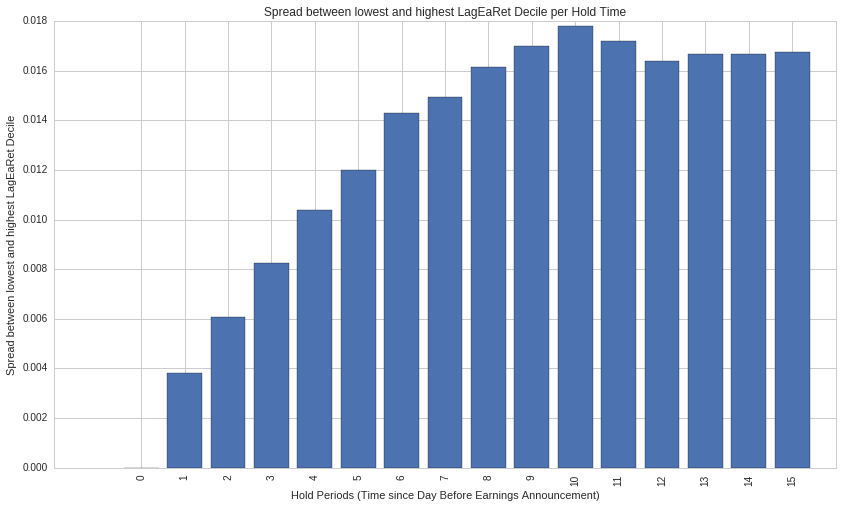In :
plot_spread_hold(stock_data, 'LagEaSurp Decile')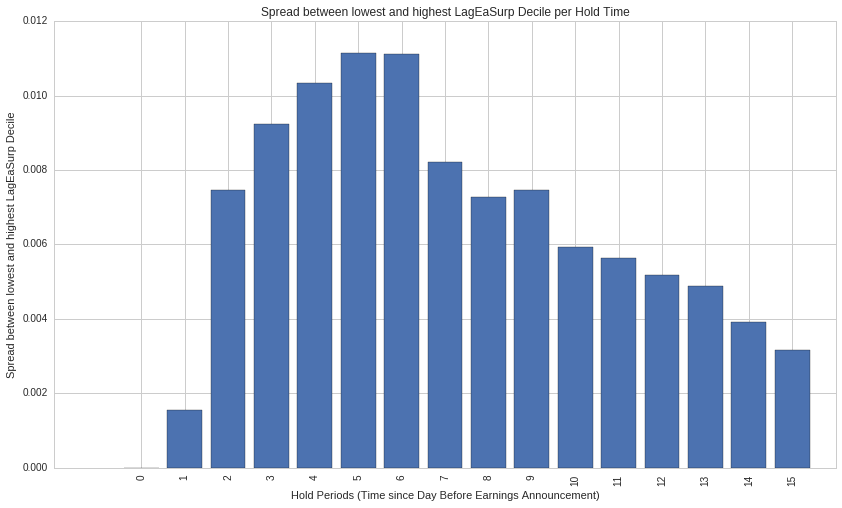There are some interesting results here. It seems that 2 days after the announcement is not the ideal time to sell for either LagEaRet and LagEaSurp. Instead , T + 10 and T + 6 after our purchase date seem to be the best times to close the position.

It's interesting that LagEaSurp tends to have a big dropoff after the 6 day mark, while LagEaRet. One could potentially use the dropoff at T + 6 to short the stocks which were previously held long and profit.

From now on, I'll use the hold period of T 10 and T 6 for subsequent results

Since the PEAD effect is based off over/underreaction to earnings news, perhaps sectors with more public exposure will have a greater susceptibility to the PEAD reversal.

We can plot the spread for each sector for both LagEaRet and LagEaSurp

In :
plot_spread_sector(stock_data, "LagEaRet Decile", 'T 10')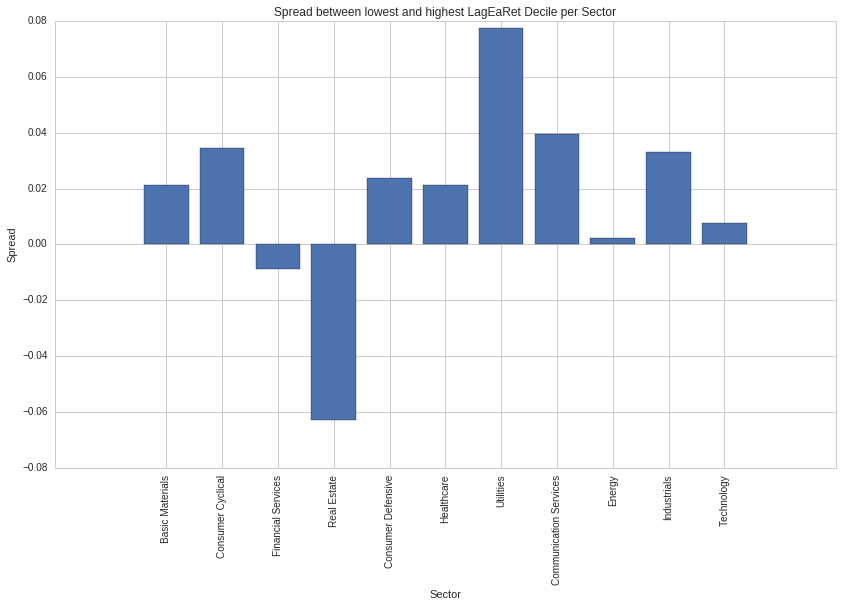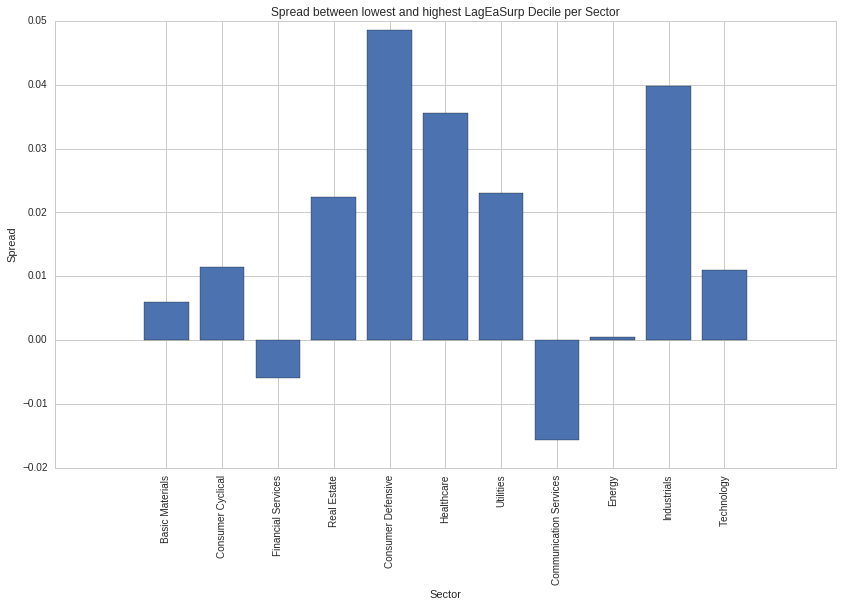Overall, no one sector seems to hold much an impact over a PEAD reversal strategy, although energy and financial services seem to be wise sectors to avoid. Perhaps with a bigger universe, we can see more constant trends between sectors

We can also look at Average Dollar Volume. The paper found that firms with active trading options are the most prone to PEAD Reversal, so perhaps high ADV companies will be more reliably responsibe to our factors.

By looking at the returns above a certain ADV floor, we can determine which ADV cutoffs seem promising.

In :
plot_spread_adv(stock_data, "LagEaRet Decile", 'T 3')

('Percentile Floor with Highest Returns', 99)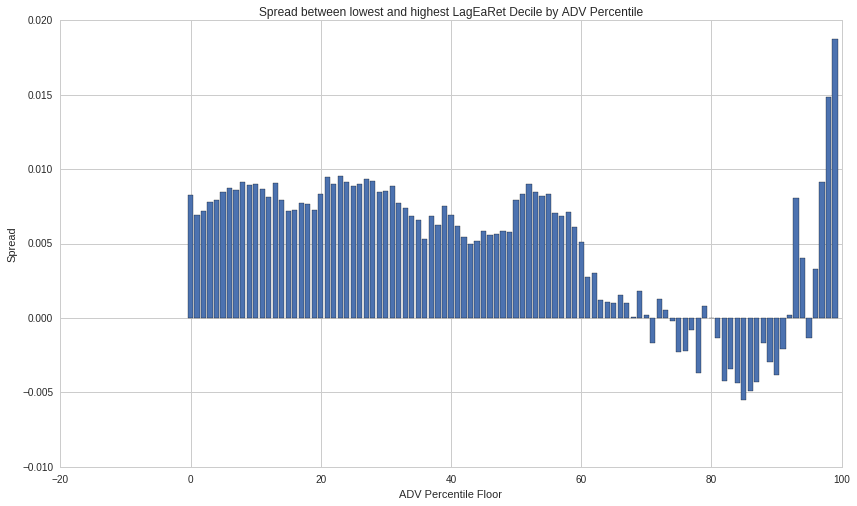('Percentile Floor with Highest Returns', 96)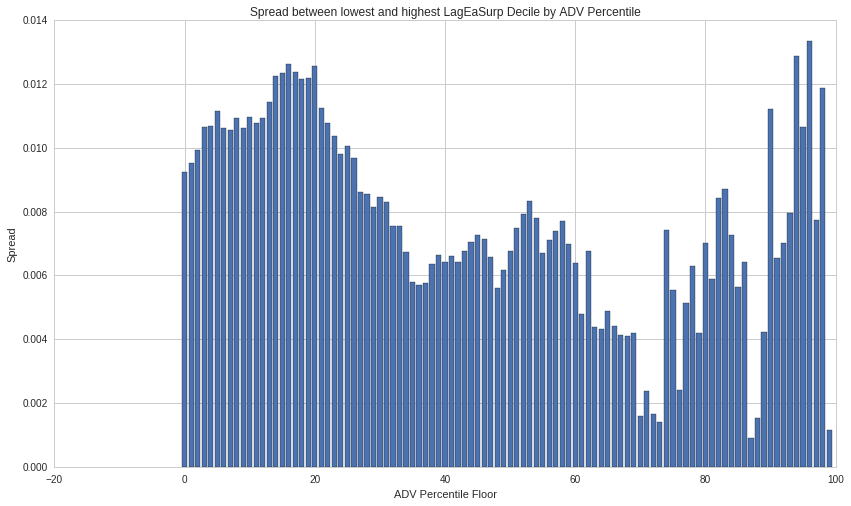It seems that this is indeed true - firms in the top 5% are consistently the highest returners for both LagEaRet and LagEaSurp

Due to the small universe of the Q500US(), we aren't able to see very much consistency using both ADV Percentile and Sector - there simply aren't enough data points. It would be interesting to expand the universe to the Q1500US or something bigger and see the ADV Percentile and Sector filters.

</a>

# Strategy Implementation¶

Let's recap the observations we've found so far:

1. Hold time is optimized at T + 10 for LagEaRet, T + 6 for LagEaSurp
2. LagEaRet is on average, a better indicator than LagEaSurp
3. Companies in the highest decile of ADV tend to have the most pronounced PEAD reversal for LagEaRet
4. Sector is mostly a non - factor

So - an ideal strategy would be:

1. Look for stocks with an Earnings announcement the next day
2. Shorting the stocks in the top decile of LagEaRet and going long on the the bottom decile of LagEaRet
3. Holding the stocks till T + 10

Until we can get our hands on a bigger universe, we will omit the ADV percentile filter as our selection of stocks will be too sparse.

We can see the results and performance of this strategy over our sample period

In :
plot_spread_year(stock_data, 'LagEaRet Decile', 'T 10')

dec_plot(stock_data, 'LagEaRet Decile', 'T 10')

low_dec_performance(stock_data, 'LagEaRet Decile', 'T 10')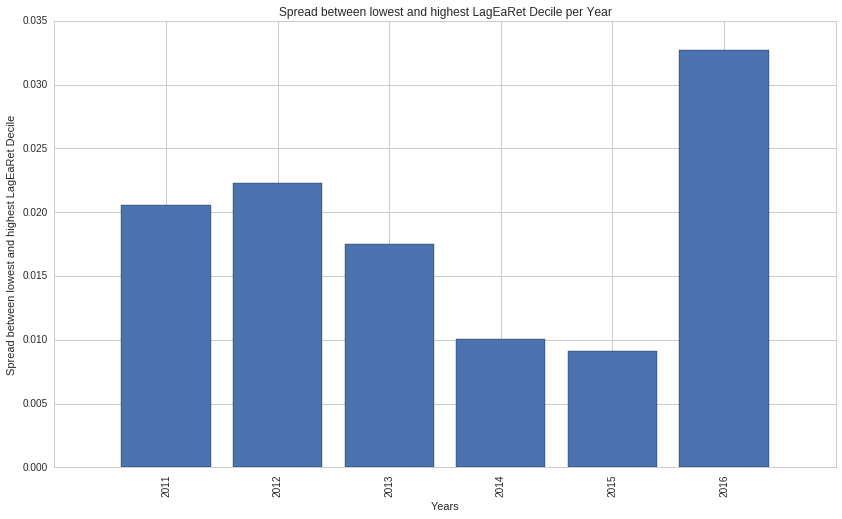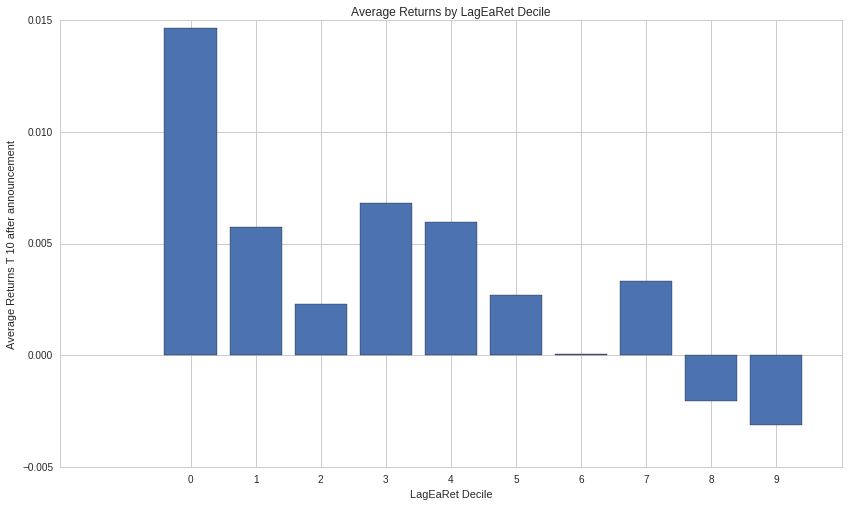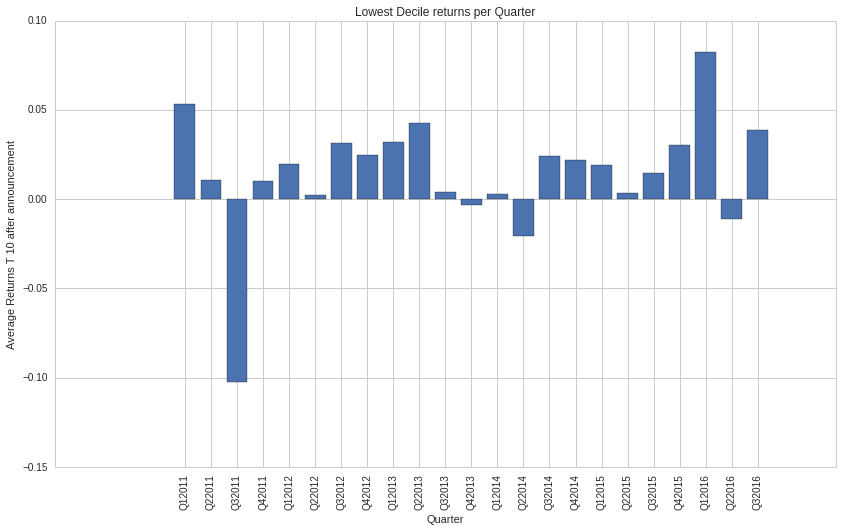In :
print ("LagEaRet 0 Decile Avg Returns", stock_data[stock_data["LagEaRet Decile"] == 0]["T 10"].mean())
print ("LagEaRet 9 Decile Avg Returns", stock_data[stock_data["LagEaRet Decile"] == 9]["T 10"].mean())
print ("LagEaRet Decile Spread Returns", stock_data[stock_data["LagEaRet Decile"] == 0]["T 10"].mean() - stock_data[stock_data["LagEaRet Decile"] == 9]["T 10"].mean())

print ("LagEaSurp 0 Decile Avg Returns", stock_data[stock_data["LagEaSurp Decile"] == 0]["T 6"].mean())
print ("LagEaSurp 9 Decile Avg Returns", stock_data[stock_data["LagEaSurp Decile"] == 9]["T 6"].mean())
print ("LagEaSurp Decile Spread Returns", stock_data[stock_data["LagEaSurp Decile"] == 0]["T 6"].mean() - stock_data[stock_data["LagEaSurp Decile"] == 9]["T 6"].mean())

('LagEaRet 0 Decile Avg Returns', 0.014662961605485464)
('LagEaRet 9 Decile Avg Returns', -0.003138852290272938)
('LagEaSurp 0 Decile Avg Returns', 0.009932191311566117)
('LagEaSurp 9 Decile Avg Returns', -0.001182087087328907)


</a>

# Conclusion¶

Overall, we found evidence of the PEAD reversal continuing over the sample period from 2011 - 2016. While we found lackluster results with the 2 day hold period recommended by the paper, the 10 day hold period with the LagEaRet indicator seemed to produce results similiar to the paper.

Milian's paper and our OOS study provide interesting insight into how well known cross sectional anomalies can reverse over time. Future work in examining other cross sectional anomalies has the potential to lead to fruitful results.

</a>

# Imports: Click and run the following cell!¶

In :
import matplotlib.pyplot as plt
import numpy as np
import pandas as pd
import math
import scipy
import bisect
import seaborn as sns
import alphalens

from quantopian.pipeline import CustomFactor, Pipeline
from quantopian.research import run_pipeline
from quantopian.pipeline.data.builtin import USEquityPricing
from quantopian.pipeline.factors import AverageDollarVolume
from quantopian.pipeline.factors import Returns
from quantopian.pipeline.filters.morningstar import Q500US, Q1500US
from quantopian.pipeline.classifiers.morningstar import Sector

from quantopian.pipeline.data.zacks import EarningsSurprises
from quantopian.pipeline.data.accern import alphaone
from quantopian.pipeline.factors.eventvestor import (
)
from quantopian.pipeline.data.eventvestor import EarningsCalendar

from odo import odo
import blaze as bz
from datetime import timedelta
from pytz import utc

TIMES =['T 0', 'T 1', 'T 2', 'T 3', 'T 4', 'T 5', 'T 6', 'T 7', 'T 8', 'T 9', 'T 10', 'T 11', 'T 12', 'T 13', 'T 14', 'T 15']

#Split so we don't go over memory limits
def split_run_pipeline(pipeline, start, end, segments):
dts = np.arange(start, end, (end - start) / segments)
if len(dts) == segments:
dts = np.append(dts, end)
return pd.concat(map(
lambda i: run_pipeline(pipeline, dts[i], dts[i + 1] - pd.Timedelta('1d')),
range(segments)
))

def run_pipeline_freq(start, end, pipeline):
'''
Runs a pipeline given a pandas datetime frequency like "Q"
'''
quarters = pd.date_range(start, end, freq='QS')
quarters = quarters.tolist()
if start not in quarters:
start = start - pd.tseries.offsets.QuarterOffset()
quarters.insert(0, start)

output = None
return pd.concat(map(
lambda i: run_pipeline(pipeline, quarters[i],quarters[i]),
range(len(quarters))
))

lag_e_surp = EarningsSurprises.eps_pct_diff_surp.latest
notnan = lag_e_surp.notnan()
date = EarningsSurprises.asof_date.latest

return Pipeline(columns= {
'LagEaSurp Decile': decile,
'LagEaSurp': lag_e_surp,
'Earnings Announcement Date' : date
},

price = USEquityPricing.close.latest
lag_ea_surp = EarningsSurprises.eps_pct_diff_surp.latest
sector = Sector()
lag_e_surp = EarningsSurprises.eps_pct_diff_surp.latest

return Pipeline(columns= {
'Current Price': price,
'Earnings Announcement': EarningsCalendar.next_announcement.latest,
'Previous Earnings Announcement': EarningsCalendar.previous_announcement.latest,
'Sector': sector,
'LagEaSurp' : lag_e_surp,
},

start = pd.Timestamp(start)
end = pd.Timestamp(end)

stock_data = split_run_pipeline(positions_pipeline, start, end, splits)
stock_data.dropna(inplace=True)
stock_data.reset_index(inplace=True)
stock_data.rename(columns= {
'level_0': 'Current Day',
'level_1': 'sid'
},
inplace=True)

stock_data.dropna(inplace=True)

decile_data = decile_data.dropna()
deciles = compute_deciles(decile_data)
stock_data['Quarter'] = [get_quarter(x) for x in stock_data['Current Day']]

for idx, row in stock_data.iterrows():
cutoffs = deciles[row['Quarter']]
lag_ea_surp = row['LagEaSurp']
dec = bisect.bisect_left(cutoffs, lag_ea_surp)
stock_data.set_value(idx, 'LagEaSurp Decile', dec)

stock_data.dropna(inplace=True)
return stock_data

def get_returns_window(price_data, sid, date, days_before, days_after):
"""
Calculates cumulative returns for a stock for a given window

Parameters
----------
price_data : pd.DataFrame
Pricing history DataFrame obtained from get_pricing. Index should
be the datetime index and sids should be columns.
sid : int or zipline.assets._assets.Equity object
Security that returns are being calculated for.
day : datetime object
Date that will be used as t=0 for cumulative return calcuations. All
returns will be calculated around this date.
days_before, days_after : int
Days before/after to be used to calculate returns for.

Returns
-------
sid_returns : pd.Series
Cumulative returns time series from days_before ~ days_after from date
"""
date = pd.Timestamp(date)
try:
date_index = price_data.index.get_loc(date)
base_price = price_data.iloc[date_index][sid]
except:
return None

end_index = date_index + days_after + 1
start_index = date_index - days_before

if end_index >= len(price_data.index) or start_index <=0:
return None

prices = price_data.iloc[start_index:end_index,:].loc[:,[sid]]
cumulative_returns = (prices[sid] - base_price) / base_price
cumulative_returns.index = range(-days_before, days_after + 1)
return cumulative_returns

def get_returns(price_data, sid, date, days_after):
"""
Calculates returns for a stock after a given days_after

Parameters
----------
price_data : pd.DataFrame
Pricing history DataFrame obtained from get_pricing. Index should
be the datetime index and sids should be columns.
sid : int or zipline.assets._assets.Equity object
Security that returns are being calculated for.
date : datetime object
Date that will be used as t=0 for cumulative return calcuations. Returns will be calculated versus
the price at this base date
days_after : int
Amount of days

Returns
-------
returns : float
The cumulative returns days_after the date
"""
date = pd.Timestamp(date)
try:
date_index = price_data.index.get_loc(date)
future_index = date_index + days_after
base_price = price_data.iloc[date_index][sid]
except:
return None

return (price_data.iloc[future_index][sid] - base_price) / base_price

def get_quarter(date):
'''
Returns a string of the form Year + Quarter, given a date
Ex. Given a string of "2014-01-01",
this method will return Q12014
'''
date = pd.Timestamp(date)
return 'Q' + str(date.quarter) + str(date.year)

def compute_deciles(decile_data):
'''
Given a DataFrame, computes the deciles for each quarter for a given field, returning
deciles a dict of the form {Quarter: Thresholds}. Ex. deciles['Q12014'] will return
[-4, 2, 4, 6, 9, 10 , 12, 13, 20, 400]
'''
decile_data = decile_data.reset_index()
quarters = decile_data['level_0'].unique()
quarterly_deciles = {}

for quarter in quarters:
quarter_str = get_quarter(quarter)
quarter_data = decile_data[decile_data['level_0'] == quarter]
deciles = []
for decile in range(0, 9):
deciles.append(quarter_data[quarter_data['LagEaSurp Decile'] == decile]['LagEaSurp'].max())

quarterly_deciles[quarter_str] = deciles

return quarterly_deciles

def low_dec_performance(stock_data, decile_col, returns_col):
quarters = stock_data['Quarter'].unique()
lowest_returns = [stock_data[(stock_data[decile_col] == 0) & (stock_data['Quarter'] == x)][returns_col].mean()
for x in quarters]
plt.bar(range(len(quarters)), lowest_returns, align='center')
plt.xticks(range(len(quarters)), quarters, rotation = "vertical")
plt.xlabel("Quarter")
plt.ylabel('Average Returns {} after announcement'.format(returns_col))
plt.title('Lowest {} returns per Quarter'.format(decile_col))
plt.show()

def dec_plot(stock_data, decile_col, returns_col):
decile_avg_returns = {}
for dec in range(0,10):
decile_avg_returns[dec] = stock_data[stock_data[decile_col] == dec][returns_col].mean()

plt.bar(range(len(decile_avg_returns)), decile_avg_returns.values(), align='center')
plt.xticks(range(len(decile_avg_returns) +1), decile_avg_returns.keys())
plt.xlabel(decile_col)
plt.ylabel(('Average Returns {} after announcement').format(returns_col))
plt.title('Average Returns by ' + decile_col)
plt.show()

years=[]
lowest_returns = []
highest_returns = []

for name, group in stock_data.groupby(stock_data['Current Day'].map(lambda x: x.year)):
years.append(name)
lowest_returns.append(group[group[decile_col] == 0][returns_col].mean())
highest_returns.append(group[group[decile_col] == 9][returns_col].mean())

spread =[low - high for low, high in zip(lowest_returns, highest_returns)]

plt.xticks(range(len(years)), years, rotation='vertical')
plt.xlabel('Years')
plt.ylabel('Spread between lowest and highest {}'.format(decile_col))
plt.title('Spread between lowest and highest {} per Year'.format(decile_col))
plt.show()

def plot_cum_rets(stock_data, decile_col):
y_values = []
x_axis = range(len(TIMES))

for name, group in stock_data.groupby(decile_col):
y_values.append([group.mean()[i] for i in TIMES])
#            [group.mean()["T 0"], group.mean()["T 1"], group.mean()["T 2"], group.mean()["T 3"]])

for idx, y_axis in enumerate(y_values):
if idx % 2 != 0 and idx != 9:
continue
plt.plot(x_axis, y_axis, label="Decile {}".format(idx))

plt.xticks(x_axis, x_axis)
plt.xlabel("Days after T - 1")
plt.ylabel("Cumulative Returns")
plt.title("Cumulative Returns by {}, Days after T - 1 (Purchase Date)".format(decile_col))
plt.legend(loc="best")
plt.show()

sector_names = {
101: 'Basic Materials',
102: 'Consumer Cyclical',
103: 'Financial Services',
104: 'Real Estate',
205: 'Consumer Defensive',
206: 'Healthcare',
207: 'Utilities',
308: 'Communication Services',
309: 'Energy',
310: 'Industrials',
311: 'Technology' ,
}

sectors = []
lowest_returns = []
highest_returns = []

for name, group in stock_data.groupby('Sector'):
sectors.append(sector_names[name])
lowest_returns.append(group[group[decile_col] == 0][returns_col].mean())
highest_returns.append(group[group[decile_col] == 9][returns_col].mean())

spread =[low - high for low, high in zip(lowest_returns, highest_returns)]

plt.xticks(range(len(sectors)), sectors , rotation='vertical')
plt.xlabel('Sector')
plt.title('Spread between lowest and highest {} per Sector'.format(decile_col))
plt.show()

lowest_returns = []
highest_returns = []

for period in TIMES:
lowest_returns.append(stock_data[stock_data[decile_col] == 0][period].mean())
highest_returns.append(stock_data[stock_data[decile_col] == 9][period].mean())

spread =[low - high for low, high in zip(lowest_returns, highest_returns)]

plt.xticks(range(len(TIMES)), range(len(TIMES)), rotation='vertical')
plt.xlabel('Hold Periods (Time since Day Before Earnings Announcement)')
plt.ylabel('Spread between lowest and highest {}'.format(decile_col))
plt.title('Spread between lowest and highest {} per Hold Time'.format(decile_col))
plt.show()

lowest_returns = []
highest_returns = []

for i in range(0,100):
group = stock_data[stock_data['ADV Percentile'] >= i]
lowest_returns.append(group[group[decile_col] == 0][returns_col].mean())
highest_returns.append(group[group[decile_col] == 9][returns_col].mean())

spread =[low - high for low, high in zip(lowest_returns, highest_returns)]

plt.show()

def compute_returns(stock_data, price_data):
# Compute LagEaRet
for idx, row in stock_data.iterrows():
prev_earnings_date = row["Previous Earnings Announcement"]
sid = row["sid"]
lagearet = get_returns(price_data, sid, pd.Timestamp(prev_earnings_date) - pd.Timedelta('1d'), 3)
stock_data.set_value(idx, 'LagEaRet', lagearet)

# Compute cumulative returns window around earnings announcement
for idx, row in stock_data.iterrows():
t = row["Current Day"]
sid = row["sid"]
returns_window = get_returns_window(price_data, sid, t, 0,  15)
if returns_window is not None:
for index, ret in returns_window.iteritems():
stock_data.set_value(idx, ('T {}').format(index), ret)

stock_data.dropna(inplace=True)

# Compute Deciles for LagEaRet
deciles = pd.Series()
for quarter in stock_data["Quarter"].unique():
quarter_data = stock_data[stock_data["Quarter"] == quarter]
deciles = deciles.append(pd.qcut(quarter_data["LagEaRet"], 10, labels= range(0,10)))

stock_data["LagEaRet Decile"] = deciles

return stock_data